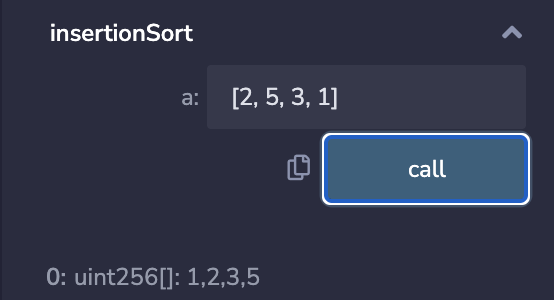# Solidity极简入门: 10. 控制流，用solidity实现插入排序

Time:
Best Score:

## 控制流​

`Solidity`的控制流与其他语言类似，主要包含以下几种：

1. `if-else`
``function ifElseTest(uint256 _number) public pure returns(bool){    if(_number == 0){    return(true);    }else{    return(false);    }}``
1. `for循环`
``function forLoopTest() public pure returns(uint256){    uint sum = 0;    for(uint i = 0; i < 10; i++){    sum += i;    }    return(sum);}``
1. `while循环`
``function whileTest() public pure returns(uint256){    uint sum = 0;    uint i = 0;    while(i < 10){    sum += i;    i++;    }    return(sum);}``
1. `do-while循环`
``function doWhileTest() public pure returns(uint256){    uint sum = 0;    uint i = 0;    do{    sum += i;    i++;    }while(i < 10);    return(sum);}``
1. `三元运算符` 三元运算符是`solidity`中唯一一个接受三个操作数的运算符，规则`条件? 条件为真的表达式:条件为假的表达式`。 此运算符经常用作 if 语句的快捷方式。
``// 三元运算符 ternary/conditional operatorfunction ternaryTest(uint256 x, uint256 y) public pure returns(uint256){    // return the max of x and y    return x >= y ? x: y; }``

## 用`solidity`实现插入排序​

### 插入排序​### `python`代码​

``# Python program for implementation of Insertion Sortdef insertionSort(arr):    for i in range(1, len(arr)):        key = arr[i]        j = i-1        while j >=0 and key < arr[j] :                arr[j+1] = arr[j]                j -= 1        arr[j+1] = key    return arr``

### 改写成`solidity`后有`BUG`！​

``    // 插入排序 错误版    function insertionSortWrong(uint[] memory a) public pure returns(uint[] memory) {                for (uint i = 1;i < a.length;i++){            uint temp = a[i];            uint j=i-1;            while( (j >= 0) && (temp < a[j])){                a[j+1] = a[j];                j--;            }            a[j+1] = temp;        }        return(a);    }``

Remix decoded output 出现错误内容### 正确的solidity插入排序​

``    // 插入排序 正确版    function insertionSort(uint[] memory a) public pure returns(uint[] memory) {        // note that uint can not take negative value        for (uint i = 1;i < a.length;i++){            uint temp = a[i];            uint j=i;            while( (j >= 1) && (temp < a[j-1])){                a[j] = a[j-1];                j--;            }            a[j] = temp;        }        return(a);    }``## 总结​Products
Community
Donation